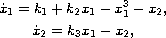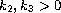Electron. J. Diff. Eqns., Vol. 2005(2005), No. 83, pp. 1-16.

### Bifurcation diagram of a cubic three-parameter autonomous system Lenka Barakova, Evgenii P. Volokitin

Abstract:
In this paper, we study the cubic three-parameter autonomous planar systemwhere. Our goal is to obtain a bifurcation diagram; i.e., to divide the parameter space into regions within which the system has topologically equivalent phase portraits and to describe how these portraits are transformed at the bifurcation boundaries. Results may be applied to the macroeconomical model IS-LM with Kaldor's assumptions. In this model existence of a stable limit cycles has already been studied (Andronov-Hopf bifurcation). We present the whole bifurcation diagram and among others, we prove existence of more difficult bifurcations and existence of unstable cycles.

Submitted February 9, 2005. Published July 19, 2005.
Math Subject Classifications: 34C05, 34D45, 34C23.
Key Words: Phase portrait; bifurcation; central manifold; topological equivalence; structural stability; bifurcation diagram;limit cycle.

Show me the PDF file (1366K), TEX file, and other files for this article.Lenka Baráková Mendel University, Dept. of Math. Zemedelska 1, 613 00 Brno, Czech Rep. email: barakova@mendelu.cz Evgenii P. Volokitin Sobolev Institute of Mathematics Novosibirsk, 630090, Russia email: volok@math.nsc.ru